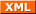#tianjara.net |Andrew Harvey's Blog

### Entries from September 2008.

29th September 2008

I always wondered why 3D points in OpenGL, Direct3D and in general computer graphics were always represented as (x,y,z,w) (i.e. why do we use four dimensions to represent a 3D point, what's the w for?). This representation of coordinates with the extra dimension is know as homogeneous coordinates. Now after finally getting formally taught linear algebra I know the answer, and its rather simple, but I'll start from the basics.

Points can be represented as vectors, eg. (1,1,1). Now a common thing we want to do in computer graphics is to move this point (translation). So we can do this by simply adding two vectors together,

$\begin{pmatrix}x'\\y'\\z'\end{pmatrix} = \begin{pmatrix}x\\y\\z\end{pmatrix} + \begin{pmatrix}a\\b\\c\end{pmatrix} = \begin{pmatrix}x + a\\y + b\\z + c\end{pmatrix}.$

If we wanted do some kind of linear transformation such as rotate about the origin, scale about the origin, etc, then we could just multiply a certain matrix with the point vector to obtain the image of the vector under that transformation. For example,

$\begin{pmatrix}x'\\ y'\\ z' \end{pmatrix} = \begin{pmatrix}\cos \theta &-\sin \theta &0\\ \sin \theta &\cos \theta &0\\ 0&0&1\end{pmatrix} \begin{pmatrix}x\\ y\\ z\end{pmatrix}$

will rotate the vector (x,y,z) by angle theta about the z axis.

However as you may have seen you cannot do a 3D translation on a 3D point by just multiplying a 3 by 3 matrix by the vector. To fix this problem and allow all affine transformations (linear transformation followed by a translation) to be done by matrix multiplication we introduce an extra dimension to the point (denoted w in this blog). Now we can perform the translation,

$\mathbb{R}^2 : (x,y) \to (x+a, y+b)$

by a matrix multiplication,

$\begin{pmatrix}1 & 0 & a\\ 0 & 1 & b\\ 0 & 0 & 1\end{pmatrix} \begin{pmatrix}x\\ y\\ 1\end{pmatrix} = \begin{pmatrix}x + a\\ y + b\\ 1 \end{pmatrix}.$

We need this extra dimension for the multiplication to make sense, and it allows us to represent all affine transformations as matrix multiplication.

REFERENCES: Homogeneous coordinates. (2008, September 29). In Wikipedia, The Free Encyclopedia. Retrieved 04:33, September 29, 2008, from http://en.wikipedia.org/w/index.php?title=Homogeneous_coordinates&oldid=241693659